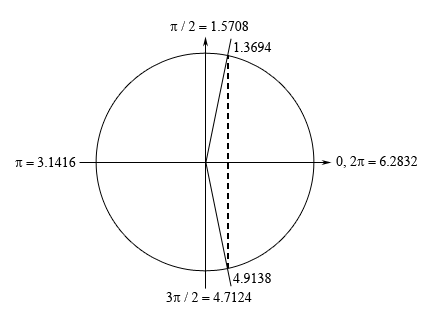Paul's Online Notes
Home / Calculus I / Review / Trig Equations with Calculators, Part I
Show Mobile Notice Show All Notes Hide All Notes
Mobile Notice
You appear to be on a device with a "narrow" screen width (i.e. you are probably on a mobile phone). Due to the nature of the mathematics on this site it is best views in landscape mode. If your device is not in landscape mode many of the equations will run off the side of your device (should be able to scroll to see them) and some of the menu items will be cut off due to the narrow screen width.

### Section 1.5 : Solving Trig Equations with Calculators, Part I

7. Find the solution(s) to $$\displaystyle 17 - 3\sec \left( {\frac{z}{2}} \right) = 2$$ that are in $$\left[ {20,45} \right]$$. Use at least 4 decimal places in your work.

Show All Steps Hide All Steps

Hint : Find all the solutions to the equation without regard to the given interval. The first step in this process is to isolate the secant (with a coefficient of one) on one side of the equation.
Start Solution

Isolating the secant (with a coefficient of one) on one side of the equation gives,

$\sec \left( {\frac{z}{2}} \right) = 5$
Hint : Using a calculator and your knowledge of the unit circle to determine all the angles in the range $$\left[ {0,2\pi } \right]$$ for which secant will have this value. The best way to do this is to rewrite the equation into one in terms of a different trig function that we can more easily deal with.
Show Step 2

In order to get the solutions it will be much easier to recall the definition of secant in terms of cosine and rewrite the equation into one involving cosine. Doing this gives,

$\sec \left( {\frac{z}{2}} \right) = \frac{1}{{\cos \left( {\frac{z}{2}} \right)}} = 5\hspace{0.25in}\hspace{0.25in}\hspace{0.25in} \Rightarrow \hspace{0.25in}\hspace{0.25in}\cos \left( {\frac{z}{2}} \right) = \frac{1}{5}$

The solution(s) to the equation involving the cosine are the same as the solution(s) to the equation involving the secant and so working with that will be easier. Using our calculator we can see that,

$\frac{z}{2} = {\cos ^{ - 1}}\left( {\frac{1}{5}} \right) = 1.3694$

Now we’re dealing with cosine in this problem and we know that the $$x$$-axis represents cosine on a unit circle and so we’re looking for angles that will have a $$x$$ coordinate of $$\frac{1}{5}$$. This means that we’ll have angles in the first (this is the one our calculator gave us) and fourth quadrant. Here is a unit circle for this situation.From the symmetry of the unit circle we can see that we can either use –1.3694 or $$2\pi - 1.3694 = 4.9138$$ for the second angle. Each will give the same set of solutions. However, because it is easy to lose track of minus signs we will use the positive angle for our second solution.

Hint : Using the two angles above write down all the angles for which cosine/secant will have this value and use these to write down all the solutions to the equation.
Show Step 3

From the discussion in the notes for this section we know that once we have these two angles we can get all possible angles by simply adding “$$+ \,2\pi n$$ for $$n = 0, \pm 1, \pm 2, \ldots$$” onto each of these.

This then means that we must have,

$\frac{z}{2} = 1.3694 + 2\pi n\hspace{0.25in}{\mbox{OR }}\hspace{0.25in}\frac{z}{2} = 4.9138 + 2\pi n\hspace{0.25in}n = 0, \pm 1, \pm 2, \ldots$

Finally, to get all the solutions to the equation all we need to do is multiply both sides by 2 and we’ll convert everything to decimals to help with the final step.

\begin{align*}z & = 2.7388 + 4\pi n& \hspace{0.25in}\,\,\,{\mbox{OR }}\hspace{0.25in} & z = 9.8276 + 4\pi n & \hspace{0.25in}n = 0, \pm 1, \pm 2, \ldots \\ & = 2.7388 + 12.5664n & \hspace{0.25in}{\mbox{OR }}\hspace{0.25in} & \hspace{0.1in}= 9.8276 + 12.5664n\hspace{0.25in}&n = 0, \pm 1, \pm 2, \ldots \end{align*}
Hint : Now all we need to do is plug in values of $$n$$ to determine which solutions will actually fall in the given interval.
Show Step 4

Now let’s find all the solutions. First notice that, in this case, if we plug in negative values of $$n$$ we will get negative solutions and these will not be in the interval and so there is no reason to even try these. Also note that if we use $$n = 0$$we will still not be in the interval and so let’s start things off at $$n = 1$$.

$\begin{array}{lclcl}{n = 1:\,}&{\require{cancel} \xcancel{{z = 15.3052}} < 20\,}& \hspace{0.25in} {{\mbox{OR}}} \hspace{0.25in} &{z = 22.3940}\\{n = 2:}&{z = 27.8716\,}& \hspace{0.25in} {{\mbox{OR}}} \hspace{0.25in} &{z = 34.9604}\\{n = 3:}&{z = 40.4380\,}& \hspace{0.25in} {{\mbox{OR}}} \hspace{0.25in} &{\xcancel{{z = 47.5268}} > 45}\end{array}$

Notice that with each increase in $$n$$ we were really just adding another 12.5664 onto the previous results and by a quick inspection of the results above we can see that we don’t need to go any farther. Also, as we’ve seen in this problem it is completely possible for only one of the solutions from a given interval to be in the given interval so don’t worry about that when it happens.

So, it looks like we have the four solutions to this equation in the given interval.

$\require{bbox} \bbox[2pt,border:1px solid black]{{z = 22.3940,\,\,27.8716,\,\,34.9604,\,\,40.4380}}$

Note that depending upon the amount of decimals you used here your answers may vary slightly from these due to round off error. Any differences should be slight and only appear around the 4th decimal place or so however.